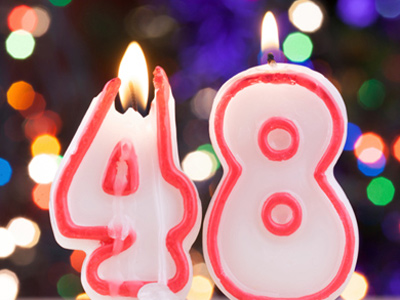48 comes exactly half way between 21 and 75.

# Compare and Order Numbers 2 (Easy)

This is the second of our easy quizzes on comparing and ordering numbers. Like the previous quiz, it will give you a chance to practice arranging amounts in ascending or descending order, and test whether or not you understand what is meant by the magnitude of a number.

This is one of the simplest things in maths. The first part should be no problem at all – as long as you can count! The second part is really simple too – assuming that you know your times tables that is. Still not sure? Then just play this quiz and you’ll soon be a master of comparing and ordering numbers.

Once you are happy with your performance in these easy quizzes, have a go at our medium ones. As is true of most things, practice makes perfect in maths. Keep testing yourself until you always score 10 out of 10!

1.
Which of the following numbers is the largest: -86, 72, 0, -72?
72
-86
-72
0
72 is the only number greater than zero, so it is the largest
2.
Starting from zero, if you keep on moving left along the number line, what happens to the numbers?
They become larger and larger positive numbers
They become smaller and smaller negative numbers
That's impossible!
They become larger and larger negative numbers
Remember that going RIGHT means positive numbers increasing. If you move LEFT starting from zero along the number line, the numbers become smaller and smaller negative numbers, not bigger and bigger
3.
How would you arrange the following numbers in descending order: 4, 14, -8, 12, 0?
-8, 0, 4, 12, 14
0, 4, -8, 12, 14
14, 12, 4, 0, -8
14, 12, -8, 4, 0
'Descending order' means that the numbers decrease in size as you read from left to right
4.
Which number lies exactly between -128 and 126?
-2
-1
0
1
Here's how you solve problems like this: add both numbers together THEN divide by two: (-128 + 126) = -2. So, -2 ÷ 2 = -1
5.
How many times bigger than 4 is 32?
4
16
12
8
32 ÷ 4= 8, so 32 is 8 times bigger than 4. That's because there are 8 lots of 4 in 32: 4 + 4 + 4 + 4 + 4 + 4 + 4 + 4 = 32
6.
How would you arrange the following numbers in ascending order: 5, 9, 2, 0, -1?
-1, 0, 2, 5, 9
9, 5, 2, 0, -1
0, -1, 2, 5, 9
9, 5, 2, -1, 0
'Ascending order' means that the numbers increase in size as you read from left to right
7.
How many times smaller than 132 is 12?
120
24
16
11
132 ÷ 12 = 11, so 132 is 11 times bigger than 12. That's because there are 11 lots of 12 in 132: 12 + 12 + 12 + 12 + 12 + 12 + 12 + 12 + 12 + 12 + 12 = 132
8.
Which of the following is the biggest number: -7, -77, 0, -777?
-777
-77
-7
0
All the other numbers are negative, so zero is the biggest number. ZERO IS A NUMBER
9.
What is wrong with this calculation: 17 + 26 - 17 = 43?
17 + 26 - 17 = 17
17 + 26 - 17 = -43
17 + 26 - 17 = 26
17 + 26 - 17 = -26
-17 + 17 = 0. Whenever you have two identical numbers that differ only in sign - they cancel each other out to give zero
10.
What number lies exactly halfway between 21 and 75?
44
46
48
50
Here's how you solve problems like this: add both numbers together THEN divide by two: (21 + 75) = 96. So, 96÷ 2 = 48
Author:  Frank Evans